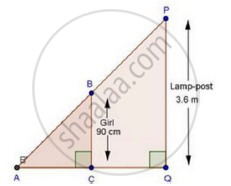Share

# A Girl of Height 90 Cm is Walking Away from the Base of a Lamp-post at a Speed of 1.2m/Sec. If the Lamp is 3.6 M Above the Ground, Find the Length of Her Shadow After 4 Seconds. - Mathematics

Course

#### Question

A girl of height 90 cm is walking away from the base of a lamp-post at a speed of 1.2m/sec. If the lamp is 3.6 m above the ground, find the length of her shadow after 4 seconds.

#### SolutionWe have,

Height of girl = 90 cm = 0.9 m

Height of lamp-post = 3.6 m

Speed of girl = 1.2 m/sec

∴ Distance moved by girl (CQ) = Speed × Time

= 1.2 × 4 = 4.8m

Let length of shadow (AC) = x cm

In ΔABC and ΔAPQ

∠ACB = ∠AQP                          [Each 90°]

∠BAC = ∠PAQ                         [Common]

Then, ΔAB ~ ΔAPQ                  [By AA similarity]

therefore"AC"/"AQ"="BC"/"PQ"                 [Corresponding parts of similar Δ are proportional]

rArrx/(x+4.8)=0.9/3.6

rArrx/(x+4.8)=1/4

⇒ 4x = x + 4.8

⇒ 4x – x = 4.8

⇒ 3x = 4.8

rArrx=4.8/3=1.6 m

∴ Length of shadow = 1.6m

Is there an error in this question or solution?

#### APPEARS IN

RD Sharma Solution for Class 10 Maths (2018 (Latest))
Chapter 7: Triangles
Ex. 7.5 | Q: 21 | Page no. 75
RD Sharma Solution for Class 10 Maths (2018 (Latest))
Chapter 7: Triangles
Ex. 7.5 | Q: 21 | Page no. 75

#### Video TutorialsVIEW ALL 

Solution A Girl of Height 90 Cm is Walking Away from the Base of a Lamp-post at a Speed of 1.2m/Sec. If the Lamp is 3.6 M Above the Ground, Find the Length of Her Shadow After 4 Seconds. Concept: Triangles Examples and Solutions.
S